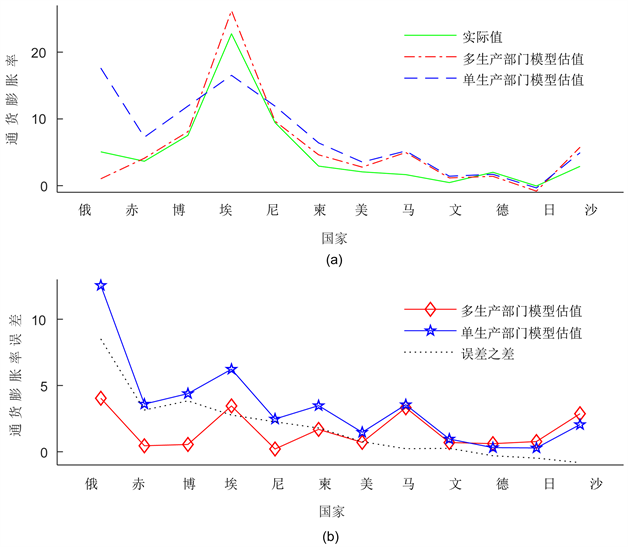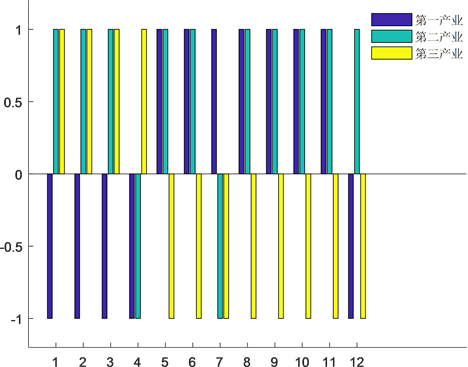# 三大产业对通货膨胀的非对称性作用及实证分析The Asymmetric Effect of Three Industries on Inflation and Empirical Analysis

• 全文下载: PDF(582KB)    PP.1-8   DOI: 10.12677/WER.2018.71001
• 下载量: 668  浏览量: 1,860   国家自然科学基金支持

This paper examines the inflation of industrial structure based on theoretical models with survey data for different economic entities. Firstly, we establish the monetary policy model of multi-production department and estimate the model parameters based on the statistical data. Then the inflation rate of the following years is predicted by the model, and is compared with the inflation rate of the single production department. The analysis shows that the forecast value of the monetary policy model of the multi-production sectors is more accurate and effective than the model of single-production department. Meanwhile, it is found that the primary industries and secondary/tertiary industries have negative and positive contribution to inflation in the economic entities with the higher proportion of primary industry, respectively. On the contrary, the tertiary industries and primary/secondary industries have negative and positive contribution to inflation in the economic entities with the higher proportion of secondary and tertiary industry, respectively. While the contributions of the secondary industry to inflation for almost economies are positive. We find that the feature of inflation possesses asymmetric effect for the three industries, and the influence form is different for economic entities with different industrial structure. This result provides some theoretical enlightenment to adjust and control inflation for government.

1. 引言

2. 模型构建及求解

2.1. 巴罗–戈登模型即单生产部门货币政策模型回顾

$M\left(x,y\right)=-c{\pi }^{2}-{\left(y-k\stackrel{¯}{y}\right)}^{2},\text{\hspace{0.17em}}c>0,\text{\hspace{0.17em}}k>1$

$y=\stackrel{¯}{y}+\beta \left(\pi -{\pi }^{\prime }\right),\text{\hspace{0.17em}}\beta >0$

$\begin{array}{l}\mathrm{max}M\left(\pi ,y\right)=-c{\pi }^{2}-{\left(y-ky\right)}^{2}\\ \text{s}\text{.t}\text{.}\text{\hspace{0.17em}}y=\stackrel{¯}{y}+\beta \left(\pi -{\pi }^{\prime }\right)\end{array}$ (1)

${\pi }^{\prime }={\pi }^{*}={c}^{-1}\beta \left(k-1\right)\stackrel{¯}{y}$ (2)

2.2. 多生产部门货币政策模型构建

$\begin{array}{l}\mathrm{max}M\left(\pi ,{y}_{1},{y}_{2},{y}_{3}\right)=-\stackrel{¯}{c}{\pi }^{2}-{\left({y}_{1}-{k}_{1}\stackrel{¯}{{y}_{1}}\right)}^{2}-{\left({y}_{2}-{k}_{2}\stackrel{¯}{{y}_{2}}\right)}^{2}-{\left({y}_{3}-{k}_{3}\stackrel{¯}{{y}_{3}}\right)}^{2}\\ \text{s}\text{.t}\text{.}\\ \text{\hspace{0.17em}}\text{\hspace{0.17em}}\text{\hspace{0.17em}}\text{\hspace{0.17em}}\text{\hspace{0.17em}}\text{\hspace{0.17em}}\text{\hspace{0.17em}}{y}_{1}=\stackrel{¯}{{y}_{1}}+{\beta }_{1}\left(\pi -{\pi }_{1}\right)\\ \text{\hspace{0.17em}}\text{\hspace{0.17em}}\text{\hspace{0.17em}}\text{\hspace{0.17em}}\text{\hspace{0.17em}}\text{\hspace{0.17em}}\text{\hspace{0.17em}}{y}_{2}=\stackrel{¯}{{y}_{2}}+{\beta }_{2}\left(\pi -{\pi }_{2}\right)\\ \text{\hspace{0.17em}}\text{\hspace{0.17em}}\text{\hspace{0.17em}}\text{\hspace{0.17em}}\text{\hspace{0.17em}}\text{\hspace{0.17em}}\text{\hspace{0.17em}}{y}_{3}=\stackrel{¯}{{y}_{3}}+{\beta }_{3}\left(\pi -{\pi }_{3}\right)\end{array}$ (3)

${\pi }^{\text{*}}={\stackrel{¯}{c}}^{-1}\left[{\beta }_{1}\left({k}_{1}-1\right){\stackrel{¯}{y}}_{1}+{\beta }_{2}\left({k}_{2}-1\right){\stackrel{¯}{y}}_{2}+{\beta }_{3}\left({k}_{3}-1\right){\stackrel{¯}{y}}_{3}\right]$ .(4)

3. 模型参数估计及相关实证分析

4. 结果分析及模型启示

Table 1. (a) The parameter estimation in multi-production departments model for different economic entities; (b) The parameter estimation in a single departments model for different economic entitiesTable 2. The predicted value and errors of inflation for multi-production and single production departments models in 2012Table 3. The average proportion of the three industries from 1996 to 2015其中，12个经济体分别指俄(罗斯联邦)、赤(道几内亚)、博(茨瓦纳)、埃(塞俄比亚)、尼(泊尔)、柬(埔寨)、美(国)、马(来西亚)、文(莱)、德(国)、日(本)、沙(特阿拉伯)。

Figure 1. The predicted value and errors of inflation for multi-production and single production departments models of different economies in 2012其中，横坐标数字1-12分别代表埃塞俄比亚、尼泊尔、沙特阿拉伯、柬埔寨、马来西亚、赤道几内亚、俄罗斯联邦、博茨瓦纳、文莱、德国、美国、日本等12个经济体。 ${\beta }_{i}>0$ 取值为1， ${\beta }_{i}<0$ 取值为−1。

Figure 2. The asymmetric effect of the three industries on inflation for different economic entities

NOTES

*通讯作者。

  徐亚平. 通货膨胀预期形成的模型刻画及其货币政策的关联性[J]. 金融研究, 2010, 363(9): 19-33.  潘敏, 缪海斌. 产业结构调整与中国通货膨胀缺口持久性[J]. 金融研究, 2012, 381(3): 14-28.  杨达. 中国货币政策的产业非对称效应[J]. 东北大学学报, 2011, 13(5): 398-403.  Ganley, J. and Chris, S. (1997) The Industrial Impact of Monetary Policy Shocks: Some Styled Facts. Bank of England, London.  张维迎. 博弈论与信息经济学[M]. 上海: 上海人民出版社, 2004.  Hayo, B. and Uhlenbrok, B. (1999) Industry Effects of Monetary Policy in Germany. University of Marburg, Marburg.  戴金平, 金永军, 陈柳钦. 货币政策的产业效应分析——基于中国货币政策的实证研究[J]. 上海财经大学学报, 2005, 7(4): 8-15.  闫红波, 王国林. 我国货币政策产业效应的非对称性研究——来自制造业的实证[J]. 数量经济技术经济研究, 2008, 25(5): 17-29.  Atanasova, C. (2003) Credit Market Imperfections and Business Cycle Dynamics: A Nonlinear Approach. Studies in Nonlinear Dynamics and Econometrics, 7, 1-22. https://doi.org/10.2202/1558-3708.1112  Mishkin, F.S. (2007) Inflation Dynamics. National Bureau of Economic Research Working Paper No. 13147. https://doi.org/10.3386/w13147